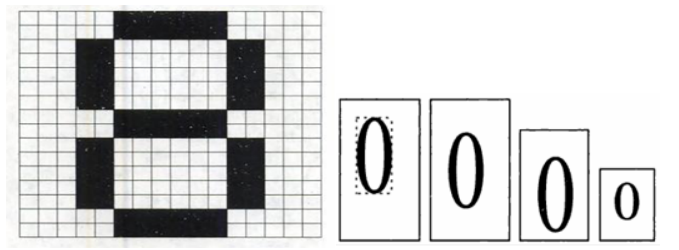﻿ 实验四：手写数字识别的神经网络算法设计与实现

### 实验四：手写数字识别的神经网络算法设计与实现

2021-11-22 12:15:23

PC机     matlab软件

# 四、实验原理

BP算法由数据流的前向计算(正向传播)和误差信号的反向传播两个过程构成. 正向传播时,传播方向为输入层→隐层→输出层,每层神经元的状态只影响下一层神经元. 若在输出层得不到期望的输出,则转向误差信号的反向传播流程. 通过这两个过程的交替进行,在权向量空间执行误差函数梯度下降策略,动态迭代搜索一组权向量,使网络误差函数达到最小值,从而完成信息提取和记忆过程.

# 五、实验步骤

1、首先对手写数字图像进行预处理,包括二值化、去噪、倾斜校正、归一化和特征提取,生成BP神经网络的输入向量m和目标向量target. 其中m选取40×10的矩阵,第1列到第10列代表0～9的数字.target为9×10的单位矩阵,每个数字在其所排顺序位置输出1,其他位置输出0.

2、然后神经网络的训练过程是识别字符的基础，直接关系到识别率的高低。输送训练样本至BP神经网络训练, 在梯度方向上反复调整权值使网络平方和误差最小。学习方法采用最速下降方法，输入结点数为16*16=256，隐层传输函数为sigmoid函数(logsig)，一个输出结点，输出传输函数为pureline(purelin),隐层结点数为sqrt(256+1)+a(a=1~10),取为25。训练完毕, 把待识别数字送BP神经网络中进行仿真测试。

# 六、实验具体过程# 七、代码实现

``````clear all;
'正在生成输入向量和目标向量，请稍等...'
for kk=0:99
p1=ones(16,16);
m=strcat(int2str(kk),'.bmp');
bw=im2bw(x,0.5);
[i,j]=find(bw==0);
imin=min(i);
imax=max(i);
jmin=min(j);
jmax=max(j);
bwl=bw(imin:imax,jmin:jmax);
rate=16/max(size(bwl));
bwl=imresize(bwl,rate); [i,j]=size(bwl);
i1=round((16-i)/2);
j1=round((16-j)/2);
p1(i1+1:i1+i,j1+1:j1+j)=bwl;
p1=-1.*p1+ones(16,16);
%以图象数据形成神经网络输入向量
for m=0:15
p(m*16+1:(m+1)*16,kk+1)=p1(1:16,m+1);
end
%形成神经网络目标向量
switch kk
case{0,10,20,30,40,50,60,70,80,90}
t(kk+1)=0;
case{1,11,21,31,41,51,61,71,81,91}
t(kk+1)=1;
case{2,12,22,32,42,52,62,72,82,92}
t(kk+1)=2;
case{3,13,23,33,43,53,63,73,83,93}
t(kk+1)=3;
case{4,14,24,34,44,54,64,74,84,94}
u            t(kk+1)=4;
case{5,15,25,35,45,55,65,75,85,95}
t(kk+1)=5;
case{6,16,26,36,46,56,66,76,86,96}
t(kk+1)=6;
case{7,17,27,37,47,57,67,77,87,97}
t(kk+1)=7;
case{8,18,28,38,48,58,68,78,88,98}
t(kk+1)=8;
case{9,19,29,39,49,59,69,79,89,99}
t(kk+1)=9;
end
end
save E52PT p t;
'输入向量和目标向量生成结束!'
``````